﻿ Buffon's Needle# Buffon's Needle

Buffon’s needle is one of the oldest problems in geometric probability. First stated in 1777 by Georges-Louis Leclerc, Comte de Buffon*, it involves dropping a needle onto a series of parallel lines (often described as a collection of parallel wooden floor boards). A remarkable outcome of this analysis is that the probabilities involved are directly related to Pi.*A prodigous naturalist, mathematician, biologist, cosmologist and author (whose works included a 44 volume encyclopaedia of the natural world). He argued for the concept of evolution and influenced Charles Darwin.

### Simple version

Imagine there are a collection of boards on the floor, each of constant width one unit. Onto these boards is dropped a needle; also of length one unit. What is the probability that the needle falls such that it lies over a crack?Below is a diagram of a needle after it falls. Since the needle is dropped entirely at random, the position can be described by two independent variables. The first is the distance d that the mid-point of needle is from the nearest edge. The second is the angle θ that needle lies relative to the parallel lines.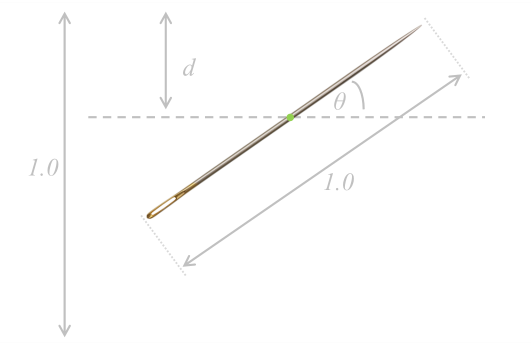In the above diagram, the needle does not cross the line. When would it cross the line?

The needle will intersect the edge if the distance from the mid-point d is less than or equal to ½sin(θ).

We can look at this graphically. The values for θ range between 0 to π (through symmetry because, if the angle is greater, it just looks like the same needle the other way around), and the distance to the nearest line d ranges between 0 and ½ (the needle can never be more than half a unit from the nearest edge).

Every (equally likely) combination of θ and d can be represented as a point on the graph below within these ranges.

Applying the constraint that d ≤ ½sin(θ), gives the region in below. The probability that the needle crosses is therefore the ratio of area of the constraint region (blue) over the total area.The total area of the solution space is ½ × π.

The area of the blue region is the definite integral of the equation ½sin(θ)The probability that the needle crosses an edge is the area under the curve divided by the total area of the rectangle.What this means is that if you repeat the experiment of dropping a needle multiple times and record the number of times it crosses a line you should be able get an approximation for π (double the number of times you drop the needle and divide this by the number of times it crosses and the answer is your approximation for π).

 e.g. If you drop a needle 100 times, and 64 times it crosses a line, the approximation would be 200/64 ≈ 3.125 (To get a more accurate answer, many more experiments would need to be performed!)Alternatively, the other way around, since we know π, we can calculate the probability that a random needle dropped will land on a crack, and this is 2/π which is approx 63.66%

### Try it out

The following applet simulates throwing needles.

The simulation can be started and stopped using the button in the lower left. There are four speeds that can toggled between. The most recently throw needle is displayed in red, and the previously thrown 254 needles are rendered in fading shades of gray.

At the top, the total number of thrown needles, those that overlap a line, and the estimate for Pi these generate is displayed.

# Generic Solution

The above solution was for the simple case of dropping a needle the same length as the separation between the lines. Let's look at a more generic solution:Imagine we have a needle of length l and the gap between lines is t. There are two cases we need to handle: The first is for a 'short' needle (a needle which l ≤ t). For a short needle, depending on the angle it falls, it either falls in a gap, or crosses one line. The second case is if the needle is 'long' (l > t), then in addition to being able to fall in a gap or cross one line, there is an angle, above which, it will cross at least one line.

The short needle case is similar to our simple problem. The probability is the ratio of the region where the needle crosses to the total solution space. The total solution space (denominator) is larger, now , and the size of the region is now l/2sin(θ). For every possible angle the needle can land in the space:This reduces down to:(You can see that when the needle is the same are the board width, and l/t=1, then the answer simplifies to the simple solution).

When the needle is long there is a little bit more complex geometry as, at certain angles, irrespective of the position of the midpoint, the needle will cross an edge from any position of the midpoint. The equation below shows the probability based on the length of the needle and width of the boards.This is a bit of a handful, so to simplify if we define the ratio of the length of the needle to the width of the board to be x (normalize it), we get a slightly simpler equation:Plotting this on a graph where the x-axis shows the normalized ratio x and the y-axis shows the probability, we get the following curve: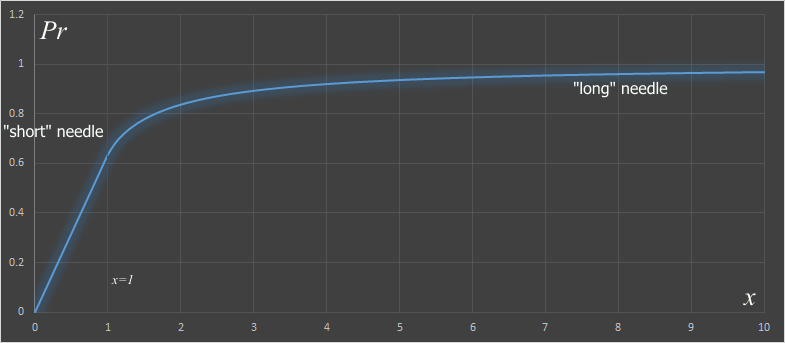When the needle is "short", the probability linearly changes with the ratio of the length (more on this later). When the needle is long, the probability asymptotes to near certainty as the the needle gets infintely longer compared to the width of the board.

# Bent needle?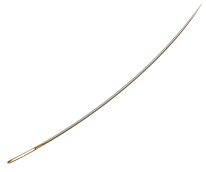What if the needle is bent? How does this affect the answer? The surprising answer is not at all! (providing that we count each of the crossings the needle makes over the edge). A bent needle is ‘shorter’ than a straight needle, so it will cross less often, but when it does cross there is a chance that it will cross the line more than once. These two things cancel out. We can prove this with a thought experiment: Imagine we have a needle of length one unit and cut it in half to make two needles of length half a unit each. We can throw both of these half-length needles on the floor. We know, from above, that the generic formula for the probability of an intersection is 2l/πt. If the two needles are half the length, the probability for each smaller element is half, and since these are independent, we just add them together to get the same original probability. Whether the two halves are connected, or not, does not matter (and if they are connected, it does not matter the angle between the two halves).

Similarly, if the needle is broken into three pieces, or four … (or at the limit an infinite number of infinitesimal pieces formed into a curve), it does not matter. All we need to do is count the number of intersections with the edge.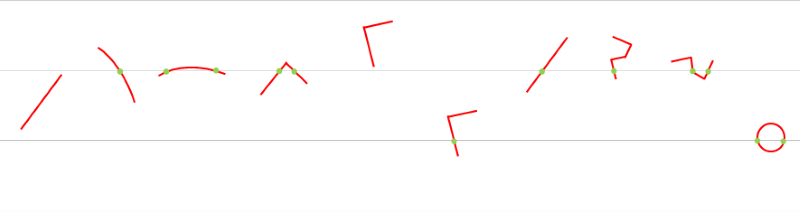It does not matter the shape of the needle, all that matters is the length of the needle (as long as the shape remains flat, and is only curved in 2D). If you threw a piece of wet spaghetti (neglecting the fact that, if it overlapped, it's not quite in the same plane), and counted the overlaps it would be the same overlap percentage as a piece of dry spaghetti of the same length. This phenomenon is sometimes given the funny descriptive name of "Buffon's Noodle!"

### Circle

Interestingly, if a circular ring of diameter the same width as the board spacing is dropped on the floor, we can prove something interesting. Because the 'length' of the ring is πt, then we can show that the it will always create two intersections. (Euclid's Elements Proposition 2).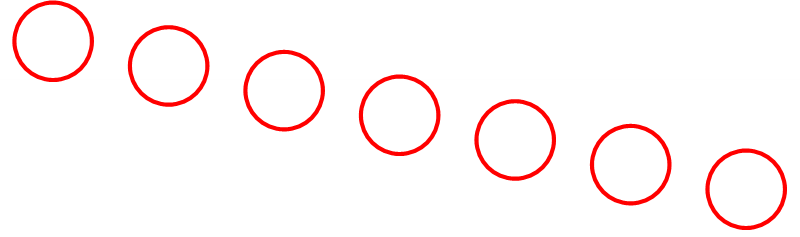### Lose the needleYou don't need to use a needle to approximate Pi. You can use any random number generator, even just a simple die, or the flip of a coin. This is called the Monte Carlo method.Using a random number generator, create a pair of coordinates and 'plot' these on a rectangular grid. Then using the equality of r2 ≤ x2+y2 for a circle, determine if the coordinate points live inside the circle. The ratio of the points that fall inside the circle compared to all point should be the same as the ratio of the area of circle to the area of the square. The diagram on the left shows an example of this using unit floating point coordinates, but the method works with quantized fixed coordinates too (such as those that could be generated by the roll of a die). The Central Limit Theorem will smooth out the answers. As is typical, however, with most Monte-Carlo simulations, convergence is painfully slow.

If a circle of radius R is inscribed inside a square with side length 2R, then the area of the circle will be πR2 and the area of the square will be (2R)2. So the ratio of the area of the circle to the area of the square will be π/4.

This means that, if you pick N points at random inside the square, approximately πN/4 of those points should fall inside the circle.

You can find a complete list of all the articles here.Click here to receive email alerts on new articles.